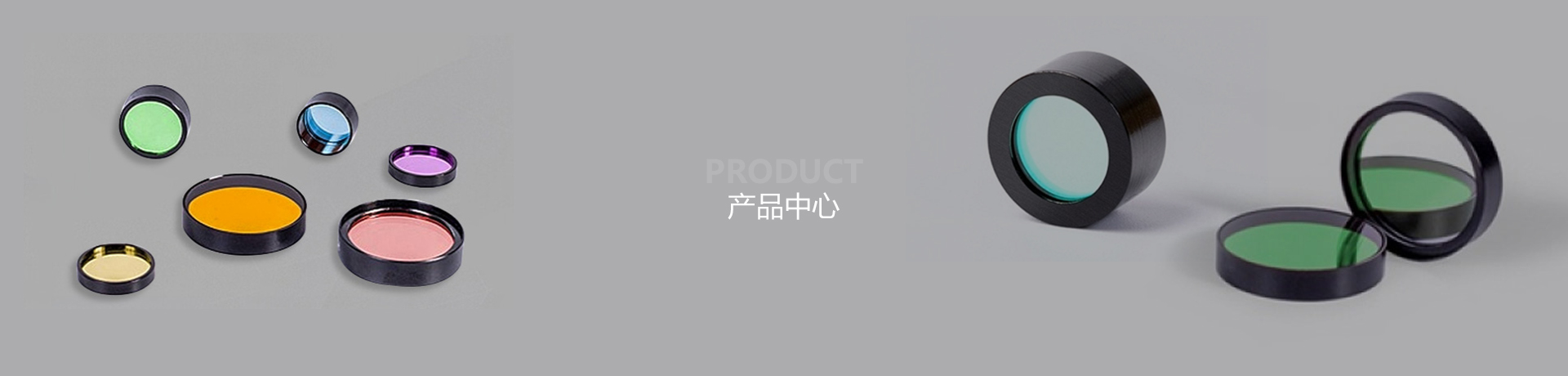# 50:50分光片（半透半反镜）

(透射比反射)T:R=1:1 (T:R=50%:50%)
T:R=60%:40%
T:R=70%:30%
T:R=80%:20%
T:R=90%:10%
T:R=95%:5%
T:R=98%:2%
R:T=99%:1%
R:T=98%:2%
R:T=95%:5%
R:T=90%:10%
R:T=80%:20%
R:T=70%:30%
R:T=60%:40%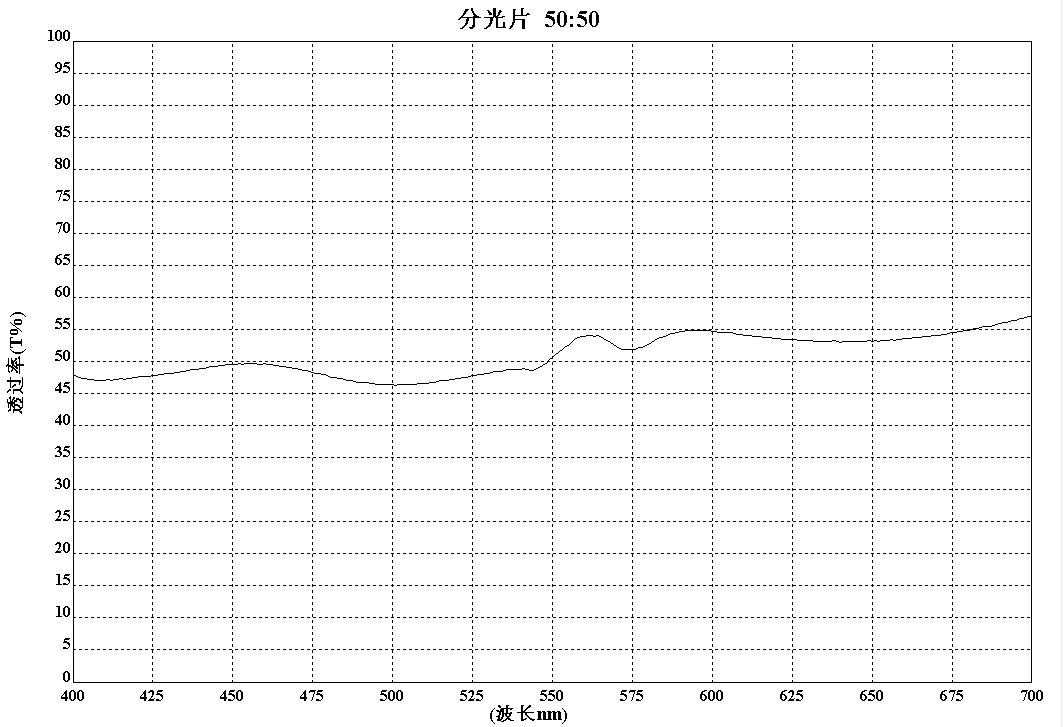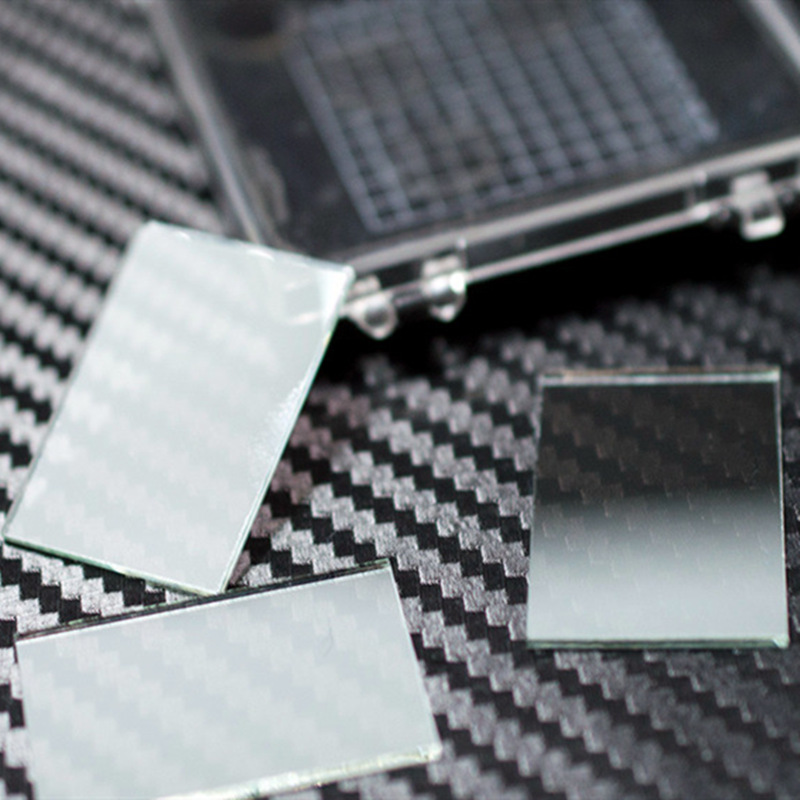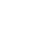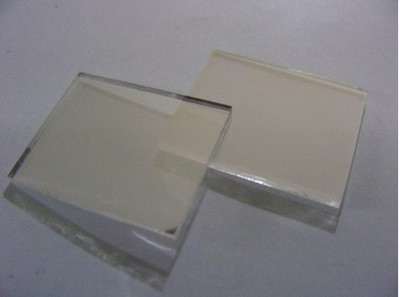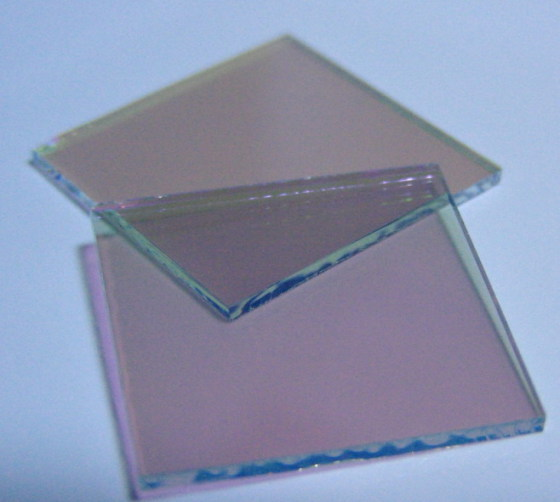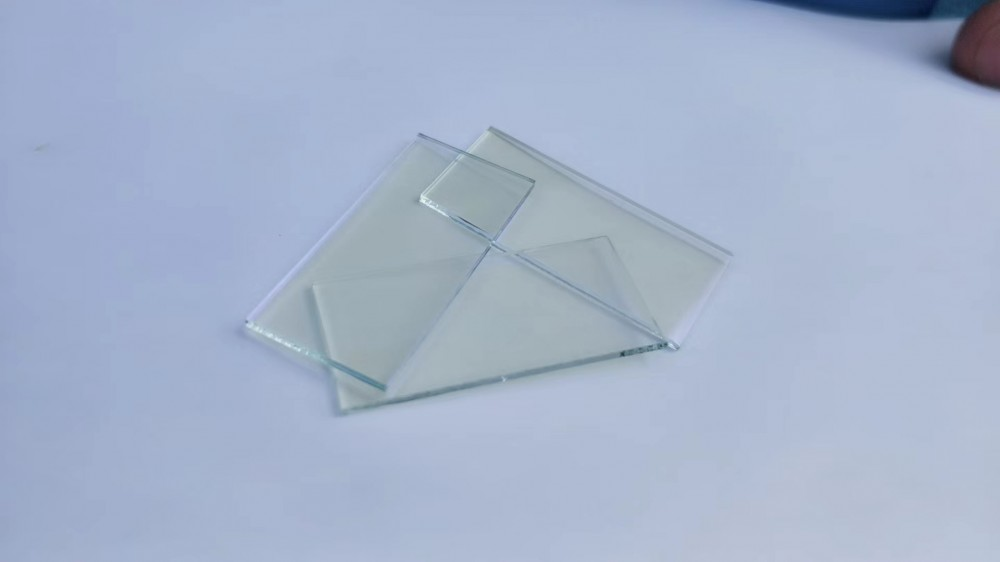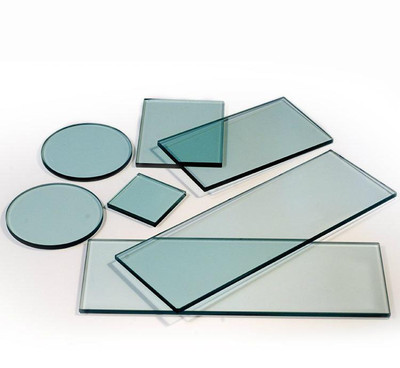•微信公众号
•微信号